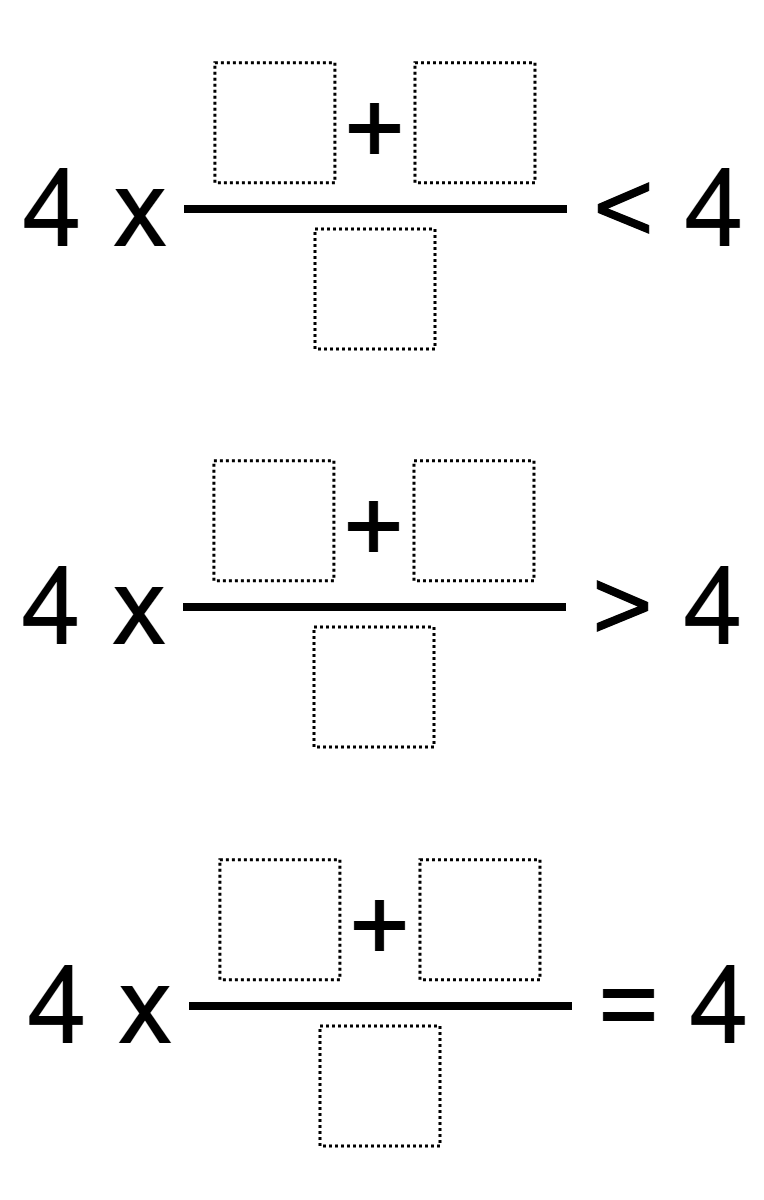# Fraction Equivalence

Directions: Using the digits 1 to 9 at most one time each, fill in the boxes to create a fraction that correctly completes each statement.### Hint

What is the result of multiplying a whole number by a proper fraction?
What is the result of multiplying a whole number by an improper fraction?